# Selina Concise Chemistry Solution for ICSE Class 10 Chapter 2 Chemical Bonding

Chemical bonding is basically defined as the attracting force between atoms, molecules or ions that enables the formation of chemical compounds. Thus, chapter 2 in Selina textbook deals with this concept in detail and it also covers all the underlying topics like the types of bonds, structure and characteristic properties of bonds and its compounds. While these are some of the main topics of the chapter, students can freely download and use concise Chemistry class 10 ICSE Solutions for chapter 2 given here to increase their understanding of the whole chapter. The solutions come with detailed explanations and answers to all questions further making it easy for students to study for tests and exams. Notably, these solutions act as a great reference tool for students and will help them to stay ahead in the class.

## Selina Concise Chemistry Solution Class 10 Chapter 2 Chemical Bonding:-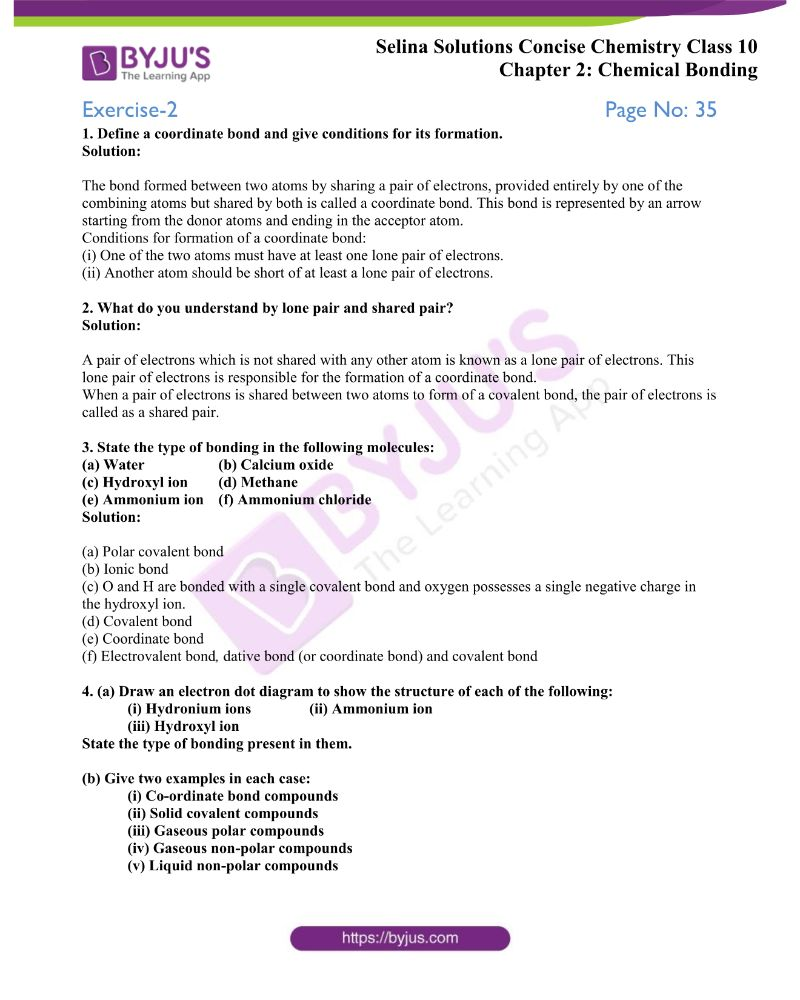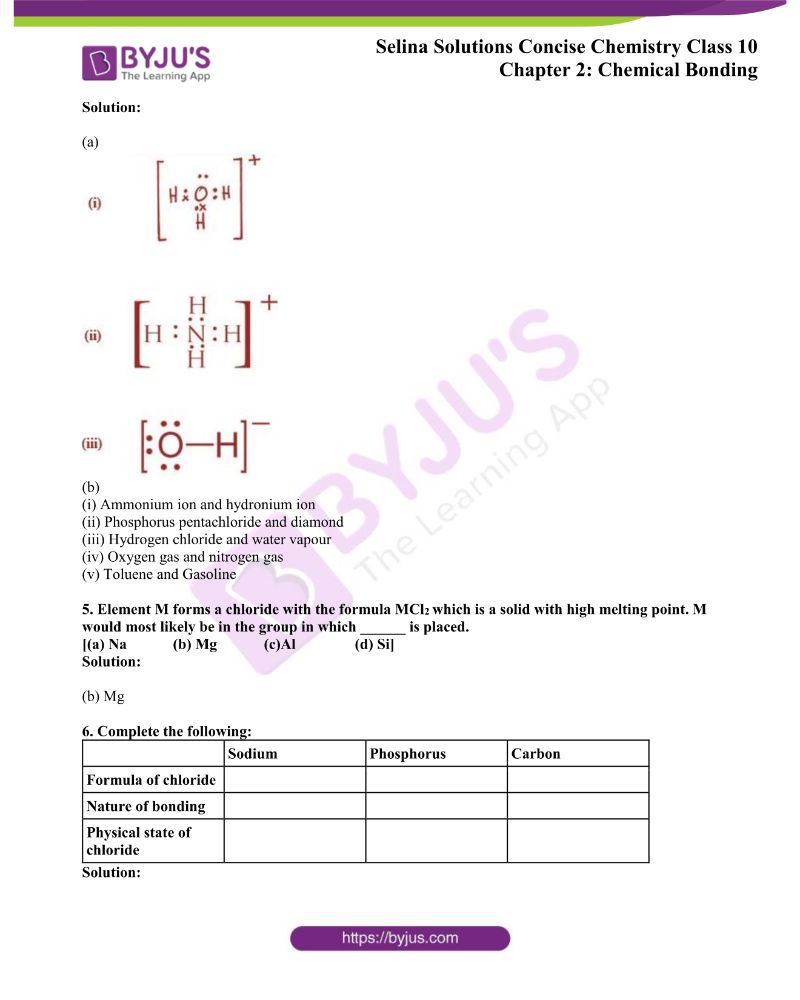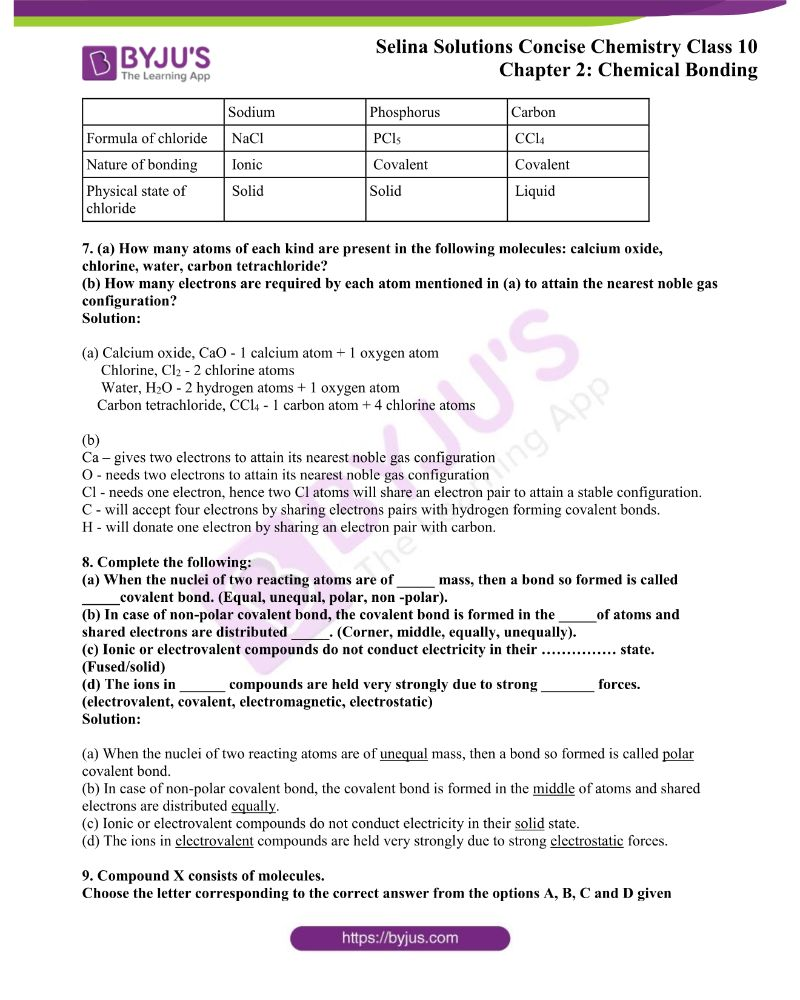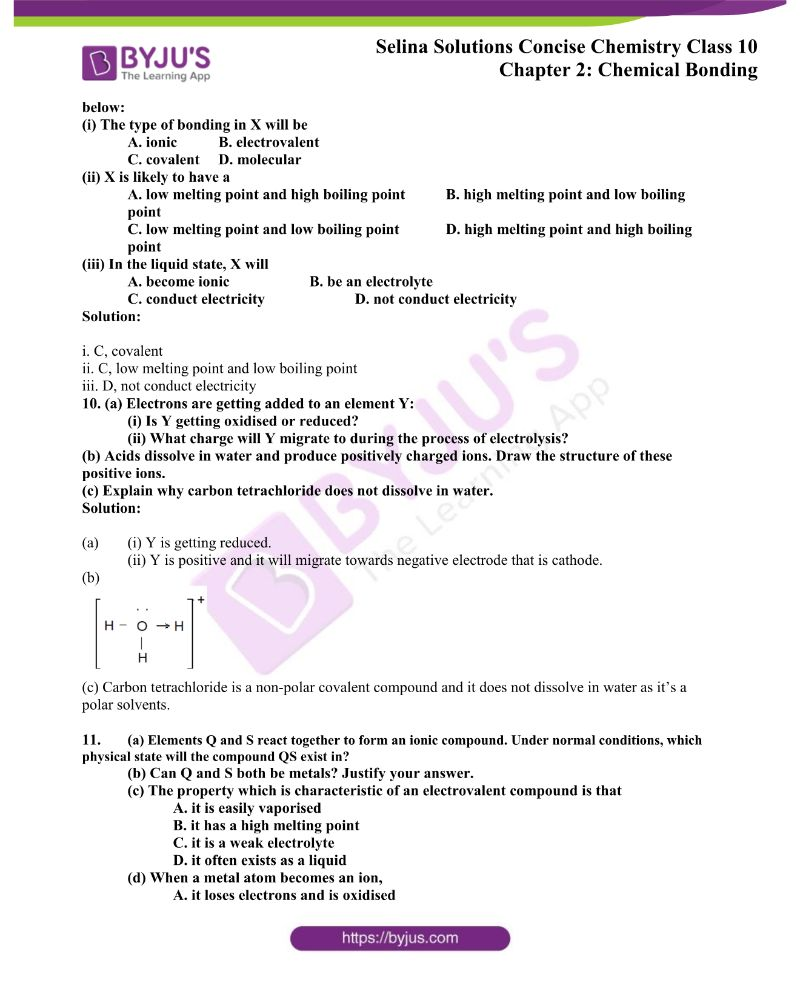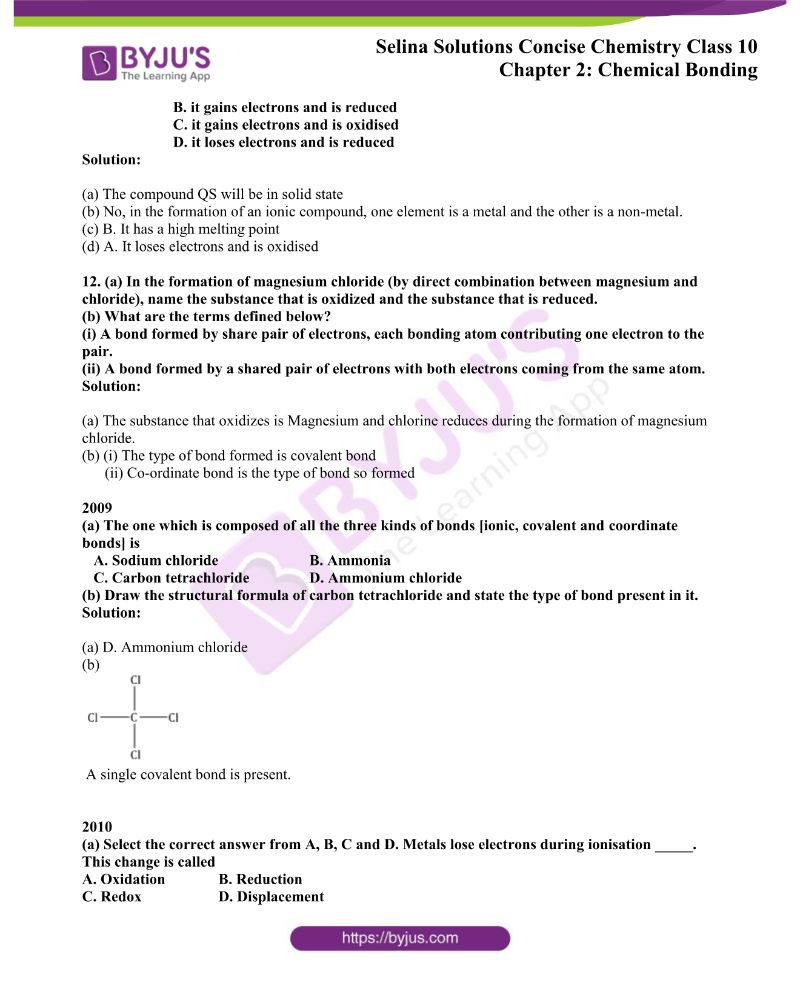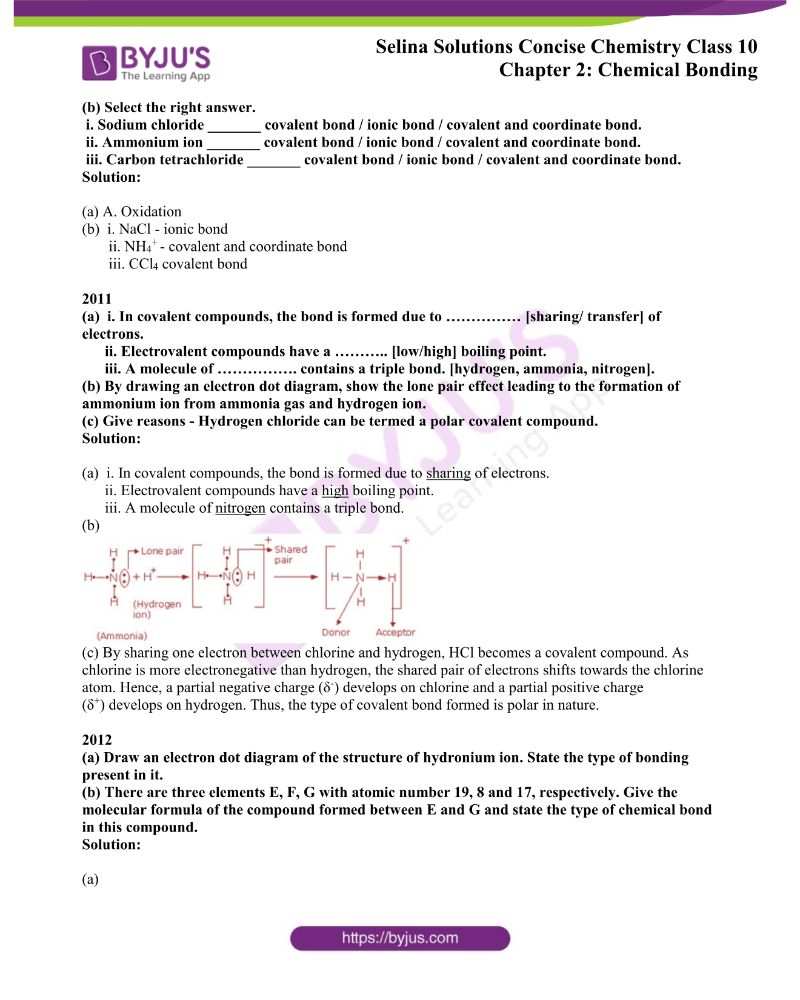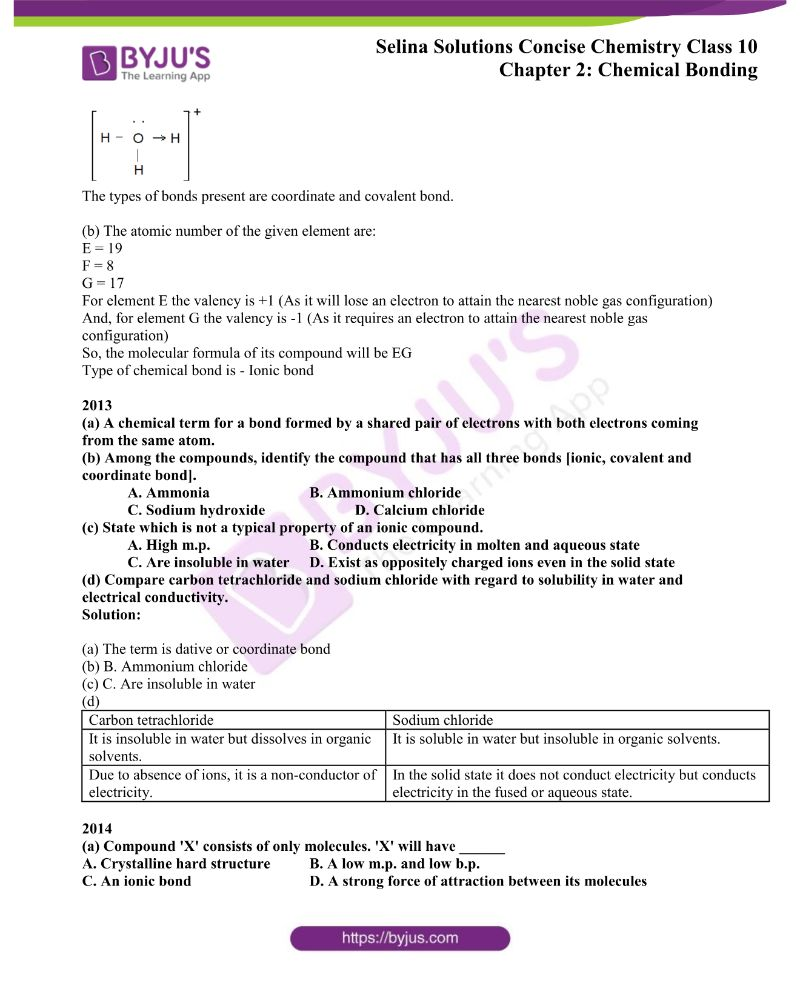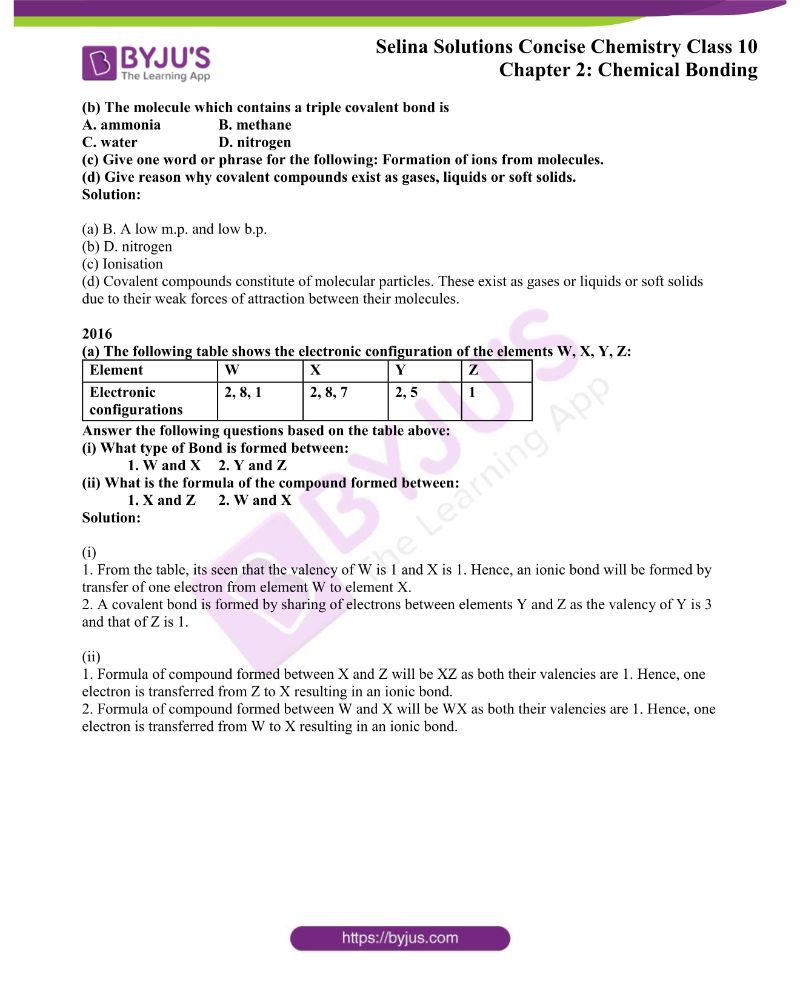### Access ICSE Selina Solutions for Class 10 Chapter 2 Chemical Bonding

Exercise-2 Page No: 35

1. Define a coordinate bond and give conditions for its formation.

Solution:

The bond formed between two atoms by sharing a pair of electrons provided entirely by one of the combining atoms but shared by both is called a coordinate bond. This bond is represented by an arrow starting from the donor atoms and ending in the acceptor atom.

Conditions for formation of a coordinate bond:

(i) One of the two atoms must have at least one lone pair of electrons.

(ii) Another atom should be short of at least a lone pair of electrons.

2. What do you understand by lone pair and shared pair?

Solution:

A pair of electrons which is not shared with any other atom is known as a lone pair of electrons. This lone pair of electrons is responsible for the formation of a coordinate bond.

When a pair of electrons is shared between two atoms to form of a covalent bond, the pair of electrons is called as a shared pair.

3. State the type of bonding in the following molecules:

(a) Water (b) Calcium oxide

(c) Hydroxyl ion (d) Methane

(e) Ammonium ion (f) Ammonium chloride

Solution:

(a) Polar covalent bond

(b) Ionic bond

(c) O and H are bonded with a single covalent bond and oxygen possesses a single negative charge in the hydroxyl ion.

(d) Covalent bond

(e) Coordinate bond

(f) Electrovalent bonddative bond (or coordinate bond) and covalent bond

4. (a) Draw an electron dot diagram to show the structure of each of the following:

(i) Hydronium ions (ii) Ammonium ion

(iii) Hydroxyl ion

State the type of bonding present in them.

(b) Give two examples in each case:

(i) Co-ordinate bond compounds

(ii) Solid covalent compounds

(iii) Gaseous polar compounds

(iv) Gaseous non-polar compounds

(v) Liquid non-polar compounds

Solution:

(a)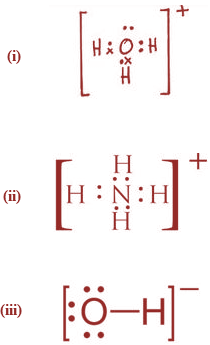(b)

(i) Ammonium ion and the hydronium ion

(ii) Phosphorus pentachloride and diamond

(iii) Hydrogen chloride and water vapour

(iv) Oxygen gas and nitrogen gas

(v) Toluene and Gasoline

5. Element M forms a chloride with the formula MClwhich is solid with a high melting point. M would most likely be in the group in which ______ is placed.

[(a) Na (b) Mg (c)Al (d) Si]

Solution:

(b) Mg

6. Complete the following:

 Sodium Phosphorus Carbon Formula of chloride Nature of bonding The physical state of chloride

Solution:

 Sodium Phosphorus Carbon Formula of chloride NaCl PCl5 CCl4 Nature of bonding Ionic Covalent Covalent The physical state of chloride Solid Solid Liquid

7. (a) How many atoms of each kind are present in the following molecules: calcium oxide, chlorine, water, carbon tetrachloride?

(b) How many electrons are required by each atom mentioned in (a) to attain the nearest noble gas configuration?

Solution:

(a) Calcium oxide, CaO – 1 calcium atom + 1 oxygen atom

Chlorine, Cl2 – 2 chlorine atoms

Water, H2O – 2 hydrogen atoms + 1 oxygen atom

Carbon tetrachloride, CCl4 – 1 carbon atom + 4 chlorine atoms

(b)

Ca – gives two electrons to attain its nearest noble gas configuration

O – needs two electrons to attain its nearest noble gas configuration

Cl – needs one electron, hence two Cl atoms will share an electron pair to attain a stable configuration.

C – will accept four electrons by sharing electrons pairs with hydrogen forming covalent bonds.

H – will donate one electron by sharing an electron pair with carbon.

8. Complete the following:

(a) When the nuclei of two reacting atoms are of _____ mass, then a bond so formed is called _____covalent bond. (Equal, unequal, polar, non -polar).

(b) In case of the non-polar covalent bond, the covalent bond is formed in the _____of atoms and shared electrons are distributed _____. (Corner, middle, equally, unequally).

(c) Ionic or electrovalent compounds do not conduct electricity in their …………… state. (Fused/solid)

(d) The ions in ______ compounds are held very strongly due to strong _______ forces. (electrovalent, covalent, electromagnetic, electrostatic)

Solution:

(a) When the nuclei of two reacting atoms are of unequal mass, then a bond so formed is called a polar covalent bond.

(b) In the case of a non-polar covalent bond, the covalent bond is formed in the middle of atoms and shared electrons are distributed equally.

(c) Ionic or electrovalent compounds do not conduct electricity in their solid-state.

(d) The ions in electrovalent compounds are held very strongly due to strong electrostatic forces.

9. Compound X consists of molecules.

Choose the letter corresponding to the correct answer from the options A, B, C and D given below:

(i) The type of bonding in X will be

A. ionic B. electrovalent

C. covalent D. molecular

(ii) X is likely to have a

A. low melting point and high boiling point B. high melting point and low boiling point

C. low melting point and low boiling point D. high melting point and high boiling point

(iii) In the liquid state, X will

A. become ionic B. be an electrolyte

C. conduct electricity D. not conduct electricity

Solution:

i. C, covalent

ii. C, low melting point and low boiling point

iii. D, not conduct electricity

10. (a) Electrons are getting added to an element Y:

(i) Is Y getting oxidised or reduced?

(ii) What charge will Y migrate to during the process of electrolysis?

(b) Acids dissolve in water and produce positively charged ions. Draw the structure of these positive ions.

(c) Explain why carbon tetrachloride does not dissolve in water.

Solution:

(a) (i) Y is getting reduced.

(ii) Y is positive and it will migrate towards a negative electrode that is a cathode.

(b)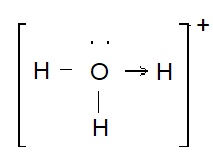(c) Carbon tetrachloride is a non-polar covalent compound and it does not dissolve in water as it’s a polar solvent.

11. (a) Elements Q and S react together to form an ionic compound. Under normal conditions, which physical state will the compound QS exist in?

(c) The property which is characteristic of an electrovalent compound is that

A. it is easily vaporised

B. it has a high melting point

C. it is a weak electrolyte

D. it often exists as a liquid

(d) When a metal atom becomes an ion,

A. it loses electrons and is oxidised

B. it gains electrons and is reduced

C. it gains electrons and is oxidised

D. it loses electrons and is reduced

Solution:

(a) The compound QS will be in solid-state

(b) No, in the formation of an ionic compound, one element is a metal and the other is a non-metal.

(c) B. It has a high melting point

(d) A. It loses electrons and is oxidised

12. (a) In the formation of magnesium chloride (by a direct combination between magnesium and chloride), name the substance that is oxidized and the substance that is reduced.

(b) What are the terms defined below?

(i) A bond formed by a shared pair of electrons, each bonding atom contributes one electron to the pair.

(ii) A bond formed by a shared pair of electrons with both electrons coming from the same atom.

Solution:

(a) The substance that oxidizes is Magnesium and chlorine reduces during the formation of magnesium chloride.

(b) (i) The type of bond formed is a covalent bond

(ii) Co-ordinate bond is the type of bond so formed

2009

(a) The one which is composed of all the three kinds of bonds [ionic, covalent and coordinate bonds] is

A. Sodium chloride B. Ammonia

C. Carbon tetrachloride D. Ammonium chloride

(b) Draw the structural formula of carbon tetrachloride and state the type of bond present in it.

Solution:

(a) D. Ammonium chloride

(b)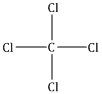A single covalent bond is present.

2010

(a) Select the correct answer from A, B, C and D. Metals lose electrons during ionisation _____. This change is called

A. Oxidation B. Reduction

C. Redox D. Displacement

i. Sodium chloride _______ covalent bond / ionic bond / covalent and coordinate bond.

ii. Ammonium ion _______ covalent bond / ionic bond / covalent and coordinate bond.

iii. Carbon tetrachloride _______ covalent bond / ionic bond / covalent and coordinate bond.

Solution:

(a) A. Oxidation

(b)  i. NaCl – ionic bond

ii. NH4+ – covalent and coordinate bond

iii. CCl4 covalent bond

2011

(a)  i. In covalent compounds, the bond is formed due to …………… [sharing/ transfer] of electrons.
ii. Electrovalent compounds have a ……….. [low/high] boiling point.

iii. A molecule of ……………. contains a triple bond. [hydrogen, ammonia, nitrogen].

(b) By drawing an electron dot diagram, show the lone pair effect leading to the formation of ammonium ion from ammonia gas and hydrogen ion.

(c) Give reasons – Hydrogen chloride can be termed a polar covalent compound.

Solution:

(a)  i. In covalent compounds, the bond is formed due to the sharing of electrons.

ii. Electrovalent compounds have a high boiling point.

iii. A molecule of nitrogen contains a triple bond.

(b)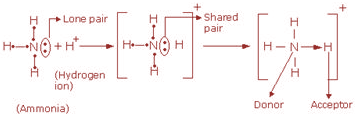(c) By sharing one electron between chlorine and hydrogen, HCl becomes a covalent compound. As chlorine is more electronegative than hydrogen, the shared pair of electrons shifts towards the chlorine atom. Hence, a partial negative charge (δ) develops on chlorine and a partial positive charge (δ+) develops on hydrogen. Thus, the type of covalent bond formed is polar in nature.

2012

(a) Draw an electron dot diagram of the structure of hydronium ion. State the type of bonding present in it.

(b) There are three elements E, F, G with atomic number 19, 8 and 17, respectively. Give the molecular formula of the compound formed between E and G and state the type of chemical bond in this compound.

Solution:

(a)The types of bonds present are coordinate and covalent bond.

(b) The atomic number of the given element is:

E = 19

F = 8

G = 17

For element E the valency is +1 (As it will lose an electron to attain the nearest noble gas configuration)

And, for element G the valency is -1 (As it requires an electron to attain the nearest noble gas configuration)

So, the molecular formula of its compound will be EG

Type of chemical bond is – Ionic bond

2013

(a) A chemical term for a bond formed by a shared pair of electrons with both electrons coming from the same atom.

(b) Among the compounds, identify the compound that has all three bonds [ionic, covalent and coordinate bond].

A. Ammonia B. Ammonium chloride

C. Sodium hydroxide D. Calcium chloride

(c) State which is not a typical property of an ionic compound.

A. High m.p. B. Conducts electricity in the molten and aqueous state

C. Are insoluble in water D. Exist as oppositely charged ions even in the solid-state

(d) Compare carbon tetrachloride and sodium chloride with regard to solubility in water and electrical conductivity.

Solution:

(a) The term is dative or coordinate bond

(b) B. Ammonium chloride

(c) C. Are insoluble in water

(d)

 Carbon tetrachloride Sodium chloride It is insoluble in water but dissolves in organic solvents. It is soluble in water but insoluble in organic solvents. Due to the absence of ions, it is a non-conductor of electricity. In the solid-state, it does not conduct electricity but conducts electricity in the fused or aqueous state.

2014

(a) Compound ‘X’ consists of only molecules. ‘X’ will have ______

A. Crystalline hard structure B. A low m.p. and low b.p.

C. An ionic bond D. A strong force of attraction between its molecules

(b) The molecule which contains a triple covalent bond is

A. ammonia B. methane

C. water D. nitrogen

(c) Give one word or phrase for the following: Formation of ions from molecules.

(d) Give a reason why covalent compounds exist as gases, liquids or soft solids.

Solution:

(a) B. A low m.p. and low b.p.

(b) D. nitrogen

(c) Ionisation

(d) Covalent compounds constitute of molecular particles. These exist as gases or liquids or soft solids due to their weak forces of attraction between their molecules.

2016

(a) The following table shows the electronic configuration of the elements W, X, Y, Z:

 Element W X Y Z Electronic configurations 2, 8, 1 2, 8, 7 2, 5 1

Answer the following questions based on the table above:

(i) What type of bond is formed between:

1. W and X  2. Y and Z

(ii) What is the formula of the compound formed between:

1. X and Z  2. W and X

Solution:

(i)

1. From the table, its seen that the valency of W is 1 and X is 1. Hence, an ionic bond will be formed by the transfer of one electron from element W to element X.

2. A covalent bond is formed by sharing of electrons between elements Y and Z as the valency of Y is 3 and that of Z is 1.

(ii)

1. The formula of the compound formed between X and Z will be XZ as both their valencies are 1. Hence, one electron is transferred from Z to X resulting in an ionic bond.

2. The formula of a compound formed between W and X will be WX as both their valencies are 1. Hence, one electron is transferred from W to X resulting in an ionic bond.

The given solutions are as per the 2019-20 Concise Selina textbook. The Selina Solutions for the academic year 2023-24 will be updated soon.